## Find the general solution of the given differential equation. 5 dy dx + 45y = 9 y(x) = Give the largest interval I over which the general so

Question

Find the general solution of the given differential equation. 5 dy dx + 45y = 9 y(x) = Give the largest interval I over which the general solution is defined. (Think about the implications of any singular points. Enter your answer using interval notation.) Determine whether there are any transient terms in the general solution.

in progress 0
5 months 2021-08-10T05:46:42+00:00 1 Answers 21 views 0

## Answers ( )

Step-by-step explanation:

Given that the differential equation is: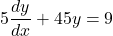by dividing both sides by 5; we have: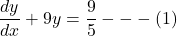which is a form of a linear equation.

The integrating factor is: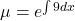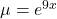multiplying eqution (1) with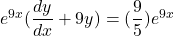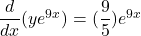Using integration on both sides.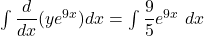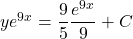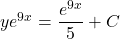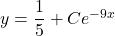Thus, the general solution is :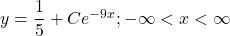In general solution: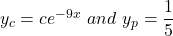To talk of larger values of x, the value of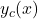is said to be negligible.

This implies that: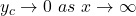Thus,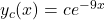signifies a transient term in the general solution.